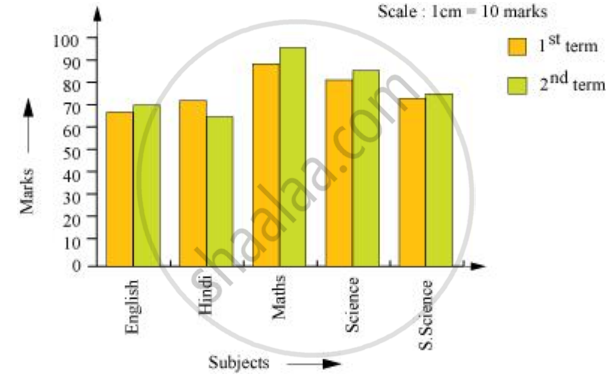Share

# The Performance of Students in 1st Term and 2nd Term is Given. Draw a Double Bar Graph Choosing Appropriate Scale and Answer the Following: - CBSE Class 7 - Mathematics

ConceptUse of Bar Graphs with a Different Purpose - Choosing a Scale

#### Question

The performance of students in 1st Term and 2nd Term is given. Draw a double bar graph choosing appropriate scale and answer the following:

 Subject English Hindi Maths Science S. science 1st Term (M.M. 100) 67 72 88 81 73 2nd Term (M.M. 100) 70 65 95 85 75

1) In which subject, has the child improved his performance the most?

2) In which subject is the improvement the least?

3) Has the performance gone down in any subject?

#### Solution

A double bar graph for the given data is as follows.1) There was a maximum increase in the marks obtained in Maths. Therefore, the child has improved his performance the most in Maths.

2) From the graph, it can be concluded that the improvement was the least in S. Science.

3) From the graph, it can be observed that the performance in Hindi has gone down.

Is there an error in this question or solution?

#### APPEARS IN

NCERT Solution for Mathematics for Class 7 (2018 to Current)
Chapter 3: Data Handling
Ex. 3.30 | Q: 4 | Page no. 73
Solution The Performance of Students in 1st Term and 2nd Term is Given. Draw a Double Bar Graph Choosing Appropriate Scale and Answer the Following: Concept: Use of Bar Graphs with a Different Purpose - Choosing a Scale.
S